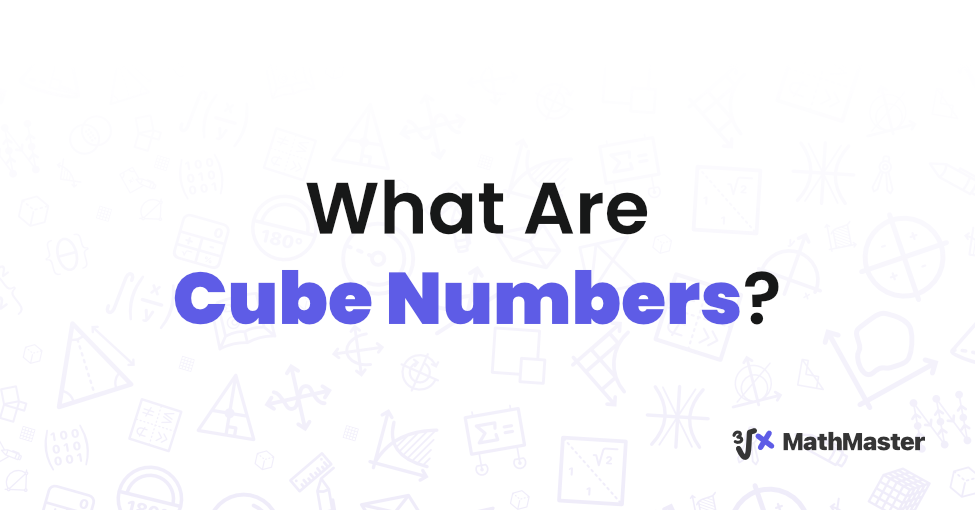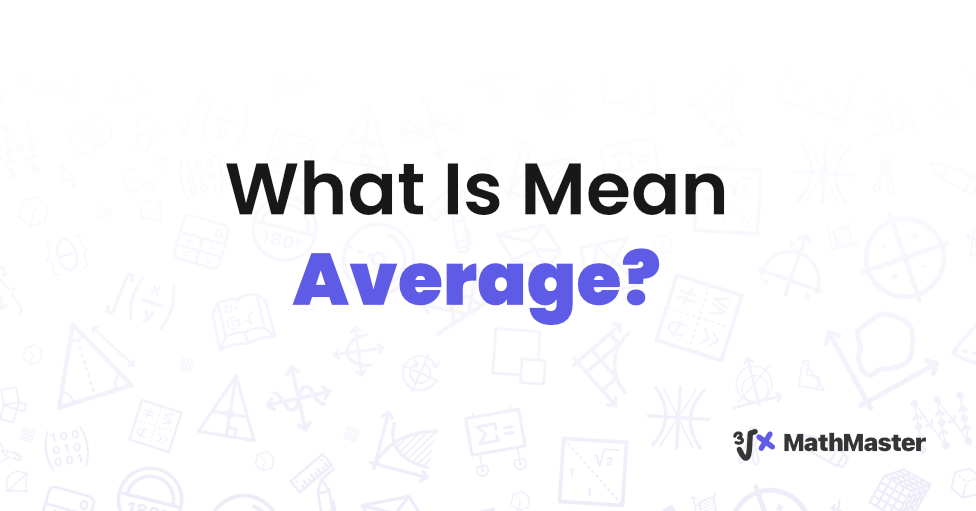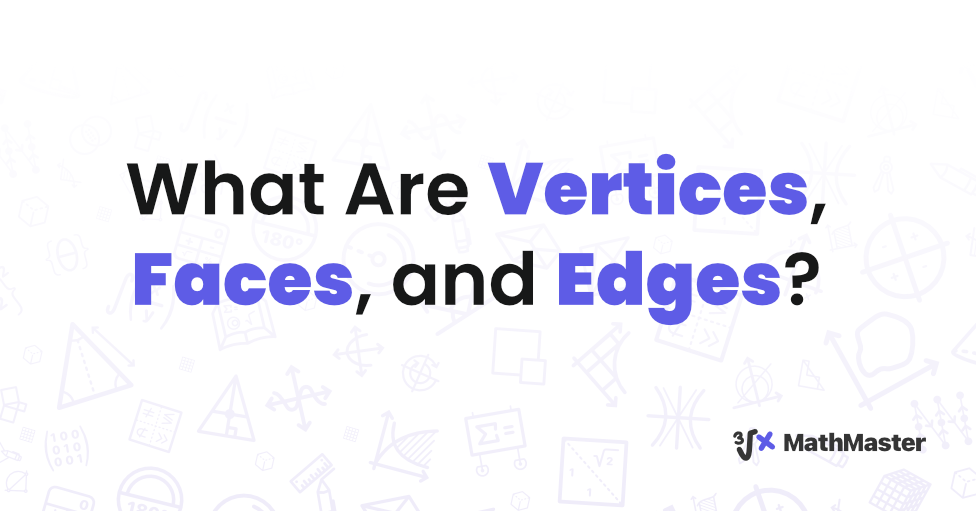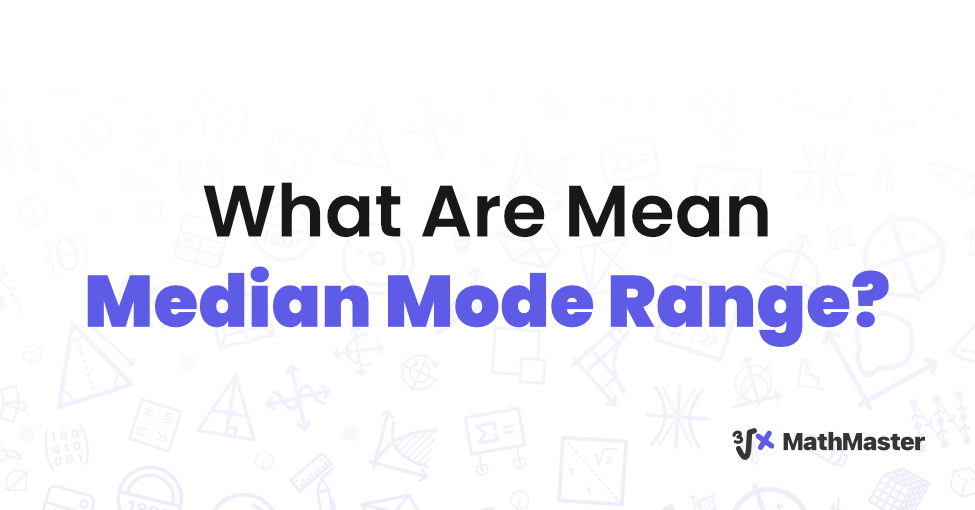# MathMaster Blog## Best AI APP For Math

Check out this ultimate list of best AI for solving math problems. With the use of these AI tools, math will be more enjoyable and easy to learn.## What are 2D shapes?

Students need to understand the concept of 2D shapes in their early learning phases.## What is a Line of Symmetry?

In mathematics, lines of symmetry are imaginary lines that are drawn through a symmetrical shape.## What Are Composite Numbers?

Numbers in mathematics are categorized into several classes. They can be whole numbers, prime numbers, rational numbers, complex numbers, composite numbers, and other categories.## What Are Cube Numbers?

In the realm of mathematics, numbers are more than mere symbols. They possess unique characteristics and properties that have intrigued mathematicians for centuries.## What Is White Rose Maths?

In the ever-evolving landscape of mathematics education, innovative approaches continue to reshape the way students learn, and teachers instruct.## What Is Mean Average?

When it comes to mathematics, one concept that frequently arises is the idea of an average.## What Is BODMAS and BIDMAS?

In the realm of mathematics, the proper order of operations is like the foundation of a building – it provides the necessary structure for complex calculations to be carried out accurately.## What Are Vertices, Faces, and Edges? A Profound Exploration of Geometric Fundamentals

In geometry, a deep understanding of vertices, faces, and edges is essential## What Are Mean Median Mode Range? Understanding Essential Statistical Concepts

Statistical concepts are crucial in the field of mathematics, as they allow individuals to analyze data effectively. Mean median mode range are fundamental terms that are essential to understand.